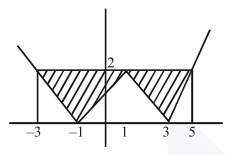# The area bounded by the lines

Question:

The area bounded by the lines $y=\| x-1|-2|$ is

Solution:

Remark :

Question is incomplete it should be area bounded by $\mathrm{y}=|\mathrm{x}-1|-2 \mid$ and $\mathrm{y}=2$Area $=2\left(\frac{1}{2} .4 .2\right)$